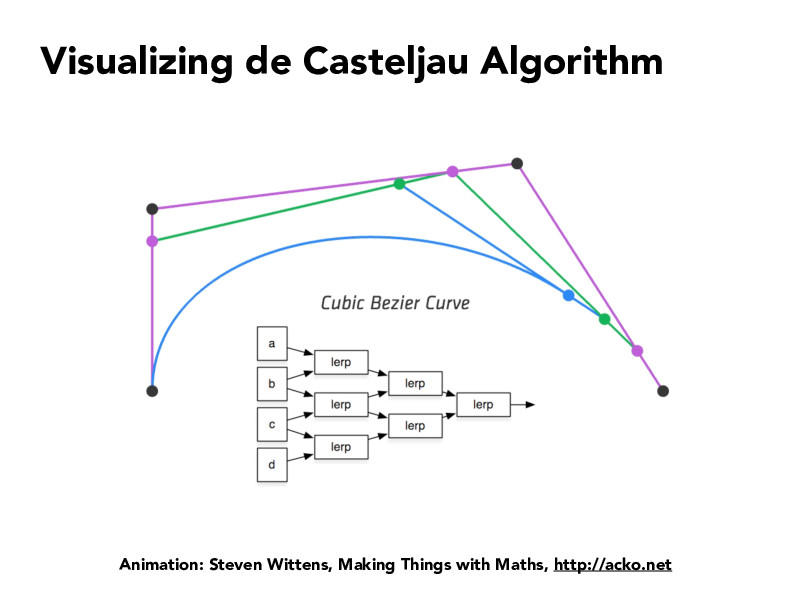Lecture 7: Splines and Bezier Curves (65)keahung

What is the advantage of using the de Casteljau Algorithm to evaluate points on the Bezier curve? I would think that it should be more efficient, but since we can combine these recursive operations into the general Bezier matrix multiplication equation, wouldn't the order of magnitude be roughly the same? The matrix multiplication will take about O(n^2) and since these recursive operations happen in a triangle shape, then the de Casteljau Algorithm would also be about O(n^2)?daniswords

I think your reasoning sounds right. I tried figuring this out too through the internet, but most of the answers I found claimed that an advantage is that the de Casteljau Algorithm is "numerically stable" (but I couldn't find explanations deeper than this/how numerical stability is defined in the context of this problem). I'm assuming that this means that the matrix version of this problem can introduce some errors while you're plugging in points, whereas the de Casteljau Algorithm will not.

You must be enrolled in the course to comment